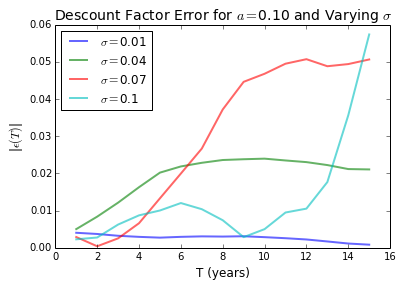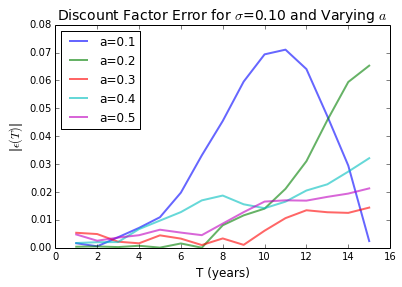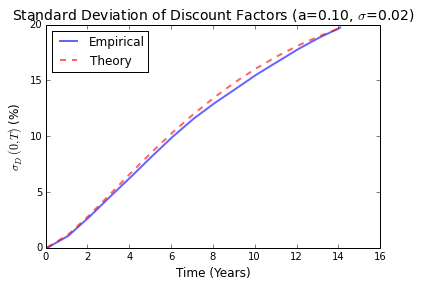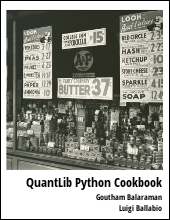Discusses the convergence of the Monte-Carlo simulations of the Hull-White model

Visit here for other QuantLib Python examples. If you found these posts useful, please take a minute by providing some feedback.

I had recently written an introductory post on simulating short rates in the Hull-White Model. This question on the QuantLib forum raised some interesting questions on the convergence of the Hull-White model simulations. In this post, I discuss the convergence of Monte-Carlo simulations using the Hull-White model.

The Hull-White Short Rate Model is defined as:

$$dr_t = (\theta(t) - a r_t)dt + \sigma dW_t$$,

where $a$ and $\sigma$ are constants, and $\theta(t)$ is chosen in order to fit the input term structure of interest rates. Here we use QuantLib to show how to simulate the Hull-White model and investigate some of the properties.

The variables used in this post are described below:

• timestep is the number of steps used to discretize the time grid
• hw_process the object that defines the Hull-White process,
• length is the time span of the simulation in years
• low_discrepancy is a boolean variable that is used to chose Sobol low discrepancy random or not
• brownnian_bridge is a boolean that choses brownian bridge for path generation
• num_paths is the number of paths in the simulation
• a is the constant parameter in the Hull-White model
• sigma is the constant parameter $\sigma$ in the Hull-White model that describes volatility
In :
Expand Code

The get_path_generator function creates the a path generator. This function takes various inputs such as

In :
Expand Code

The generate_paths function uses the generic path generator produced by the get_path_generator function to return a tuple of the array of the points in the time grid and a matrix of the short rates generated.

In :
Expand Code

The generate_paths_zero_price essentially is a wrapper around generate_path_generator and generate_paths taking all the required raw inputs. This function returns the average of zero prices from all the paths for different points in time. I wrote this out so that I can conveniently change all the required inputs and easily plot the results.

In :
Expand Code

## Factors affecting the convergence

In order to understand the convergence of Monte-Carlo for the Hull-White model, let us compare the market discount factor,

$$P^M(t, T) = \exp\left(-\int_t^Tf^{M} (t,u)du\right)$$

with the expectation of the discount factors from the sample of Monte-Carlo paths,

$$P^{MC}(t,T) = E_t\left\{ e^{-\int_t^T r^{MC}(u) du} \right\}$$.

Here $f^{M}(t, T)$ is the instantaneous forward rate implied by the market, and $r^{MC}(s)$ is the instantaneous short rate from the Monte-Carlo simulations. The error in the Monte-Carlo simulation can be defined as:

$$\epsilon(T) = P^M(0,T) - P^{MC}(0,T)$$

As a first step, let us look at the plots of $\epsilon(t)$ for different values of $a$ and $\sigma$.

In :
Expand CodeThe above plot illustrates that for $\sigma=0.01$, the Monte-Carlo model shows good convergence, and the convergence gradually deteriorates as $\sigma$ increases and approaches $a$.

In :
Expand CodeThe above plot illustrates that for $a=0.1$ the convergence of Monte-Carlo is poor, and it gradually improves as $a$ increases more than $\sigma$.

From the plots above, we observe that the convergence is good if the ratio $\sigma/a < 1$, and the convergence detiorates as the ratio $\sigma/a$ increases above unity. Now, let us try to formalize this observation from the theoretical footing of the Hull-White model.

## Distribution of Discount Factors

The Monte-Carlo approach estimates the market discount factor as the expectation of discount factors from each Monte-Carlo path. If distribution of discount factors has a standard deviation $\sigma_D$, then the error in our estimate of $P^{MC}(t,T)$ on using $N$ paths will be of the order of: $$\epsilon(t,T) \approx \frac{\sigma_D}{\sqrt(N)}.$$

In other words, there are two factors at play in our Monte-Carlo estimate, the number of Monte-Carlo paths $N$ and the standard deviation of the distribution of discount factors $\sigma$. Using more Monte-Carlo paths will lead to improved convergence. But at the same time, the $\sigma_D$ has to be relatively small for us to get a good estimate.

The integral of short rates can be shown to be normally distributed (refer Brigo-Mercurio, second edition page 75), and is given as $$\int_t^T r(u) du | \mathcal{F}_t \sim \mathcal{N}\left(B(t,T)[r(t)-\alpha(t)] + \ln\frac{P^M(0,t)}{P^M(0,T)} + \frac{1}{2}[V(0,T) - V(0,t)], V(t,T)\right)$$

where, \begin{eqnarray} B(t,T) &=& \frac{1}{a} \left[ 1 - e^{-a(T-t)}\right] \\ V(t,T) &=& \frac{\sigma^2}{a^2}\left[ T - t + \frac{2}{a}e^{-a(T-t)} - \frac{1}{2a}e^{-2a(T-t)} - \frac{3}{2a}\right] \end{eqnarray}

Based on this result, the discount factor from the Monte-Carlo simulation of short rates

$$P^{MC}(t, T) = \exp\left(- \int_t^T r(u) du | \mathcal{F}_t \right)$$

will have a log-normal distribution with a standard deviation

$$\sigma_D(t,T) = P^M(t,T)\sqrt{e^{V(t,T)} -1 }$$

This result follows from the fact that if $X$ is a random process with a normal distribution having mean $\mu$ and standard deviation $\sigma$, then log-normal distribution $Y=e^X$ will satisfy: \begin{eqnarray} E(Y) &=& e^{\mu+\sigma^2/2}\\ Var(Y) &=& (e^{\sigma^2} -1 )E(Y)^2 \end{eqnarray}

In :
Expand CodeThe plot above compares the standard deviation of the discount factors $\sigma_D$ from the closed form expression with a Monte-Carlo estimate. The empirical estimate is in agreement with the theoretical expectation. We can estimate the value of $\sigma_D$ for the asymptotic limit of $T\rightarrow\infty$:

$$\sigma_D(0, T) \approx P^M(0,T)e^{f^M(0,T)/a-\sigma^2/(4a^3)}\sqrt{e^{\sigma^2T/a^2} - 1}$$

The exponential term, $e^{\sigma^2T/a^2}$, can become very large when $\sigma^2T/a^2$ grows above 1. Thus we can expect good convergence when $\sigma^2T/a^2$ remains small or close to zero for the time $T$ of interest to us.

The above result suggests that if the parameters $\sigma$ and $a$ are not chosen carefully, (i.e. $\sigma/a >1$) then the convergence of the simulation would be poor and the results untrustworthy.

# Conclusion

This post shows how convergence of Monte Carlo simulations of Hull-White short rate model is dependent on the ratio $\sigma /a$. You can download the ipython notebook on convergence of Monte Carlo simulations of Hull-White model.

quantlib   python   finance

Related PostI am Goutham Balaraman, and I explore topics in quantitative finance, programming, and data science. You can follow me @gsbalaraman.

Checkout my bookUpdated posts from this blog and transcripts of Luigi's screencasts on YouTube is compiled into QuantLib Python Cookbook .